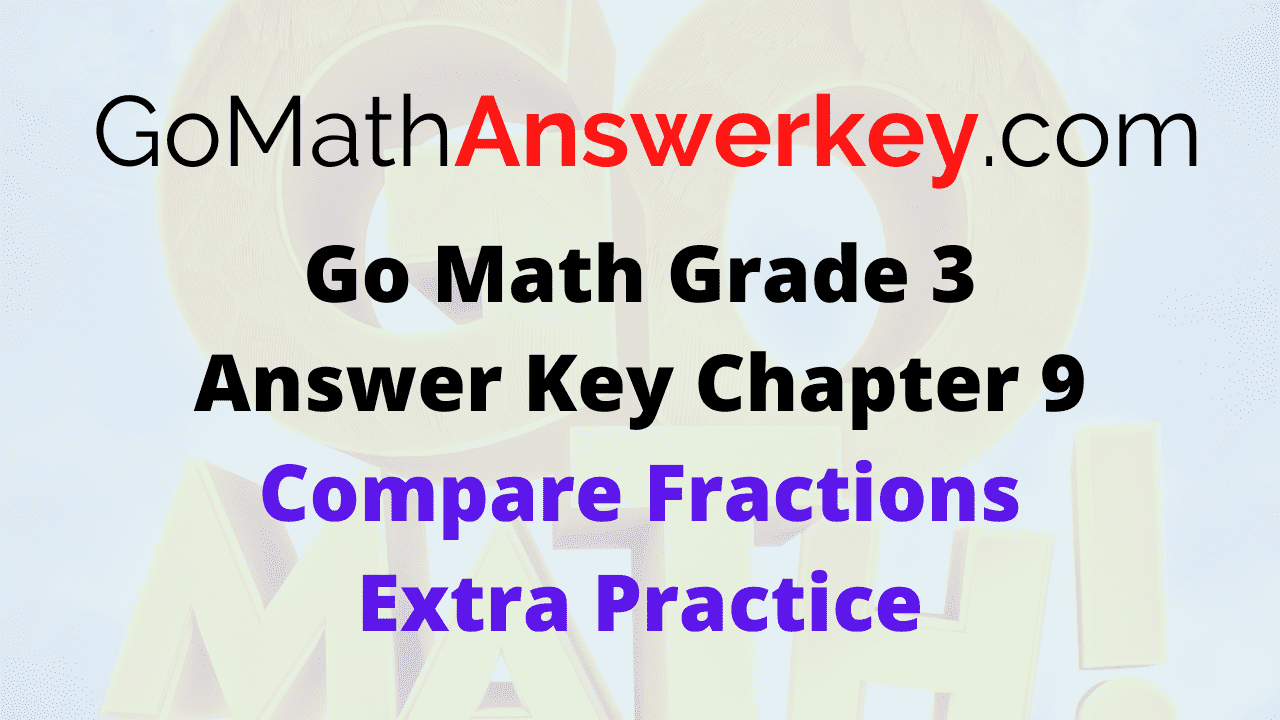# Go Math Grade 3 Answer Key Chapter 9 Compare Fractions Extra Practice

Get the Answer Key for Go Math Grade 3 Chapter 9 Compare Fractions Extra Practice here. The students who have completed exercise and homework problems can check the Go Math Grade 3 Answer Key Chapter 9 Compare Fractions Extra Practice. We provide the Question and answers along with the detailed explanation in 3rd Grade Go Math Chapter 9 Compare Fractions Extra Practice Solution Key.

## Go Math Grade 3 Answer Key Chapter 9 Compare Fractions Extra Practice

All you have to do is to check out the topics covered in this chapter before you start the preparation. There are many methods to solve the problems in compare fractions. So, Make use of links provided to understand the concepts of fractions. Solve the Questions given in the extra practice and check the solutions in the Go Math Answer Key Grade 3 Chapter 9 Compare Fractions.

### Common Core – Page No. 189000

Lesson 9.1

Question 1.
Nick finished $$\frac{4}{8}$$ of his homework before dinner. Ed finished $$\frac{7}{8}$$ of his homework before dinner. Who finished the greater part of his homework?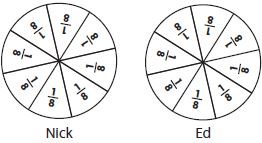_____

Explanation:

Compare the fractions $$\frac{4}{8}$$ and $$\frac{7}{8}$$
The denominator of both the fractions is the same. So, compare the numerators.
The numerator with the greatest number will be the greatest fraction.
7 is greater than 4.
$$\frac{7}{8}$$ > $$\frac{4}{8}$$
Therefore Ed finished the greater part of his homework.

Question 2.
Rafael walked $$\frac{2}{3}$$ mile and then rode his scooter $$\frac{2}{6}$$ mile. Which distance is farther?$$\frac{□} {□}$$ mile is farther

Answer: $$\frac{2}{3}$$

Explanation:

Rafael walked $$\frac{2}{3}$$ mile and then rode his scooter $$\frac{2}{6}$$ mile.
The numerator of both the fractions is the same but the denominators are different.
The fraction is smaller if the denominator is greater.
Thus $$\frac{2}{3}$$ > $$\frac{2}{6}$$
$$\frac{2}{3}$$ mile is farther.

Lessons 9.2–9.3

Compare. Write <, >, or =.

Question 3.
$$\frac{2}{6}$$ _____ $$\frac{3}{6}$$

Answer: $$\frac{2}{6}$$ < $$\frac{3}{6}$$

Explanation:

Compare the fractions $$\frac{2}{6}$$ and $$\frac{3}{6}$$
The denominators are the same and the numerators are different.
So compare the numerators of two fractions.
2 is less than 3.
So, $$\frac{2}{6}$$ < $$\frac{3}{6}$$

Question 4.
$$\frac{6}{8}$$ _____ $$\frac{1}{8}$$

Answer: $$\frac{6}{8}$$ > $$\frac{1}{8}$$

Explanation:

Compare $$\frac{6}{8}$$ and $$\frac{1}{8}$$
The denominators are the same and the numerators are different.
6 is greater than 1.
$$\frac{6}{8}$$ > $$\frac{1}{8}$$

Question 5.
$$\frac{3}{8}$$ _____ $$\frac{3}{4}$$

Answer: $$\frac{3}{8}$$ < $$\frac{3}{4}$$

Explanation:

Compare the fractions $$\frac{3}{8}$$ and $$\frac{3}{4}$$
The numerators are the same and denominators are different.
Compare the denominators of two fractions.
The fraction with lesser number will be the greatest.
$$\frac{3}{8}$$ < $$\frac{3}{4}$$

Question 6.
$$\frac{1}{6}$$ _____ $$\frac{1}{8}$$

Answer: $$\frac{1}{6}$$ > $$\frac{1}{8}$$

Explanation:

The numerator of both the fractions is the same.
The denominator with the greatest number will be the smallest fraction.
So, $$\frac{1}{6}$$ > $$\frac{1}{8}$$

Question 7.
$$\frac{2}{3}$$ _____ $$\frac{2}{6}$$

Answer: $$\frac{2}{3}$$ > $$\frac{2}{6}$$

Explanation:

The numerator of both the fractions is the same.
The denominator with the greatest number will be the smallest fraction.
$$\frac{2}{3}$$ > $$\frac{2}{6}$$

Question 8.
$$\frac{1}{8}$$ _____ $$\frac{3}{8}$$

Answer: $$\frac{1}{8}$$ < $$\frac{3}{8}$$

Explanation:

The denominator of both the fractions is the same.
So, compare the numerators. The fraction with the small number will be the smallest fraction.
$$\frac{1}{8}$$ < $$\frac{3}{8}$$

Lesson 9.4

Compare. Write <, >, or = . Write the strategy you used.

Question 9.
$$\frac{2}{8}$$ _____ $$\frac{2}{3}$$

Answer: $$\frac{2}{8}$$ < $$\frac{2}{3}$$

Explanation:

The numerator of both the fractions is the same.
Compare the denominators.
The denominator with the greatest number will be the smallest fraction.
$$\frac{2}{8}$$ < $$\frac{2}{3}$$

Question 10.
$$\frac{5}{6}$$ _____ $$\frac{1}{6}$$

Answer: $$\frac{5}{6}$$ > $$\frac{1}{6}$$

Explanation:

The denominator of both the fractions is the same.
The fraction with the small number will be the smallest fraction.
5 is greater than 1.
$$\frac{5}{6}$$ > $$\frac{1}{6}$$

Question 11.
$$\frac{7}{8}$$ _____ $$\frac{3}{4}$$

Answer: $$\frac{7}{8}$$ > $$\frac{3}{4}$$

Explanation:

Compare $$\frac{7}{8}$$ and $$\frac{3}{4}$$
Make the denominators equal to compare the fractions.
$$\frac{3}{4}$$ × $$\frac{8}{8}$$ = $$\frac{24}{32}$$
$$\frac{7}{8}$$ × $$\frac{4}{4}$$ = $$\frac{28}{32}$$
$$\frac{28}{32}$$ > $$\frac{24}{32}$$
$$\frac{7}{8}$$ > $$\frac{3}{4}$$

### Common Core – Page No. 190000

Lesson 9.5

Write the fractions in order from greatest to least.

Question 1.
$$\frac{1}{2}, \frac{1}{4}, \frac{1}{3}$$
Type below:
__________

Answer: $$\frac{1}{2}, \frac{1}{3}, \frac{1}{4}$$

Explanation:

The numerator of the three fractions is the same.
So, the order from greatest to least is $$\frac{1}{2}, \frac{1}{3}, \frac{1}{4}$$

Question 2.
$$\frac{4}{6}, \frac{1}{6}, \frac{2}{6}$$
Type below:
__________

Answer: $$\frac{4}{6}, \frac{2}{6}, \frac{1}{6}$$

Explanation:

The denominator of the three fractions is the same.
Compare the numerator of the fraction.
4 > 2 > 1
$$\frac{4}{6}, \frac{2}{6}, \frac{1}{6}$$

Question 3.
$$\frac{3}{6}, \frac{3}{4}, \frac{3}{8}$$
Type below:
__________

Answer: $$\frac{3}{4}, \frac{3}{6}, \frac{3}{8}$$

Explanation:

The numerator of the three fractions is the same.
So, the order is $$\frac{3}{4}, \frac{3}{6}, \frac{3}{8}$$

Question 4.
$$\frac{6}{8}, \frac{3}{8}, \frac{5}{8}$$
Type below:
__________

Answer: $$\frac{6}{8}, \frac{5}{8}, \frac{3}{8}$$

Explanation:

The denominator of the three fractions is the same.
Compare the numerator and write the order from greatest to least fraction.
$$\frac{6}{8}, \frac{5}{8}, \frac{3}{8}$$

Lessons 9.6–9.7

Shade the model. Then divide the pieces to find the equivalent fraction.

Question 5.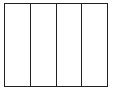$$\frac{1}{4}=\frac{■}{8}$$
$$\frac{1}{4}$$ = $$\frac{□} {□}$$

Answer: $$\frac{1}{4}$$ = $$\frac{2} {8}$$

Explanation: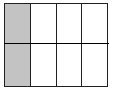$$\frac{1}{4}$$ = $$\frac{2} {8}$$

Question 6.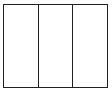$$\frac{2}{3}=\frac{■}{6}$$
$$\frac{2}{3}$$ = $$\frac{□} {□}$$

Answer: $$\frac{2}{3}$$ = $$\frac{4} {6}$$

Explanation: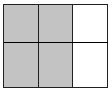$$\frac{2}{3}$$ = $$\frac{4} {6}$$

Use the number line to find the equivalent fraction.

Question 7.$$\frac{1}{2}=\frac{■}{8}$$
$$\frac{1}{2}$$ = $$\frac{□} {□}$$

Answer: $$\frac{1}{2}$$ = $$\frac{4} {8}$$

Explanation: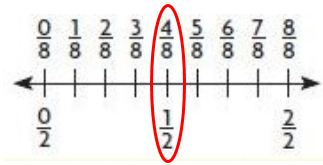Question 8.$$\frac{2}{2}=\frac{■}{6}$$
$$\frac{2}{2}$$ = $$\frac{□} {□}$$

Answer: $$\frac{2}{2}$$ = $$\frac{6} {6}$$

Explanation:Each shape is 1 whole. Shade the model to find the equivalent fraction.

Question 9.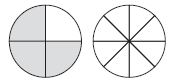$$\frac{3}{4}=\frac{■}{8}$$
$$\frac{3}{4}$$ = $$\frac{□} {□}$$

Answer: $$\frac{3}{4}$$ = $$\frac{6} {8}$$

Explanation:$$\frac{3}{4}$$ = $$\frac{6} {8}$$

Question 10.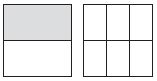$$\frac{1}{2}=\frac{■}{6}$$
$$\frac{1}{2}$$ = $$\frac{□} {□}$$

Answer: $$\frac{1}{2}$$ = $$\frac{3} {6}$$

Explanation: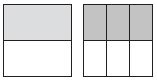$$\frac{1}{2}$$ = $$\frac{3} {6}$$

Conclusion

Early childhood mathematics is vitally important for young children’s present and future educational success. So start learning the basics from now to become master in Math. For your better understanding we have provided the answers in the form of pictures. Refer Go Math Grade 3 Answer Key Chapter 9 Extra Practice and score well in the exams. For any qrieries in extra practice go through the Go Math Grade 3 Answer Key Chapter 9 Compare Fractions pdf.

Scroll to Top